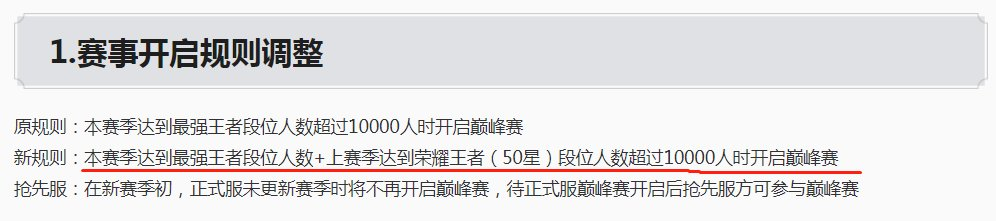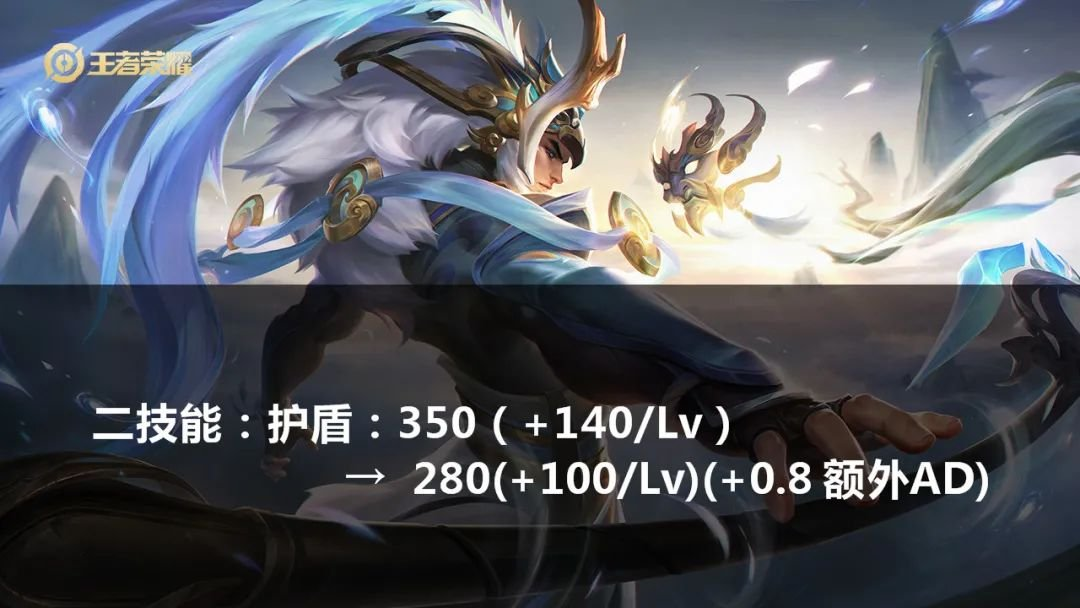20.3.2022/王者荣耀350+140*5=1050100+30+180+90+30=430攻击力9*（1+2）=27

280+（100*5）+0.8*（430+27）=1145.6

1050＜1145.6350+140=490

280+100+0.8*（30+30+27）=449.6

490＞449.6